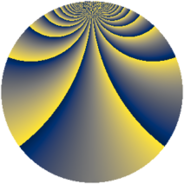# Properties

 Label 983.2.cLevel $983$ Weight $2$ Character orbit 983.c Rep. character $\chi_{983}(2,\cdot)$ Character field $\Q(\zeta_{491})$ Dimension $39690$ Newform subspaces $1$ Sturm bound $164$ Trace bound $0$

# Related objects

## Defining parameters

 Level: $$N$$ $$=$$ $$983$$ Weight: $$k$$ $$=$$ $$2$$ Character orbit: $$[\chi]$$ $$=$$ 983.c (of order $$491$$ and degree $$490$$) Character conductor: $$\operatorname{cond}(\chi)$$ $$=$$ $$983$$ Character field: $$\Q(\zeta_{491})$$ Newform subspaces: $$1$$ Sturm bound: $$164$$ Trace bound: $$0$$

## Dimensions

The following table gives the dimensions of various subspaces of $$M_{2}(983, [\chi])$$.

Total New Old
Modular forms 40670 40670 0
Cusp forms 39690 39690 0
Eisenstein series 980 980 0

## Trace form

 $$39690 q - 489 q^{2} - 487 q^{3} - 565 q^{4} - 485 q^{5} - 477 q^{6} - 489 q^{7} - 485 q^{8} - 558 q^{9} + O(q^{10})$$ $$39690 q - 489 q^{2} - 487 q^{3} - 565 q^{4} - 485 q^{5} - 477 q^{6} - 489 q^{7} - 485 q^{8} - 558 q^{9} - 471 q^{10} - 477 q^{11} - 463 q^{12} - 483 q^{13} - 473 q^{14} - 461 q^{15} - 543 q^{16} - 481 q^{17} - 457 q^{18} - 473 q^{19} - 449 q^{20} - 449 q^{21} - 459 q^{22} - 473 q^{23} - 405 q^{24} - 552 q^{25} - 437 q^{26} - 451 q^{27} - 455 q^{28} - 455 q^{29} - 379 q^{30} - 459 q^{31} - 437 q^{32} - 447 q^{33} - 423 q^{34} - 439 q^{35} - 459 q^{36} - 475 q^{37} - 423 q^{38} - 439 q^{39} - 413 q^{40} - 455 q^{41} - 407 q^{42} - 457 q^{43} - 413 q^{44} - 397 q^{45} - 415 q^{46} - 467 q^{47} - 393 q^{48} - 524 q^{49} - 429 q^{50} - 403 q^{51} - 391 q^{52} - 431 q^{53} - 379 q^{54} - 415 q^{55} - 371 q^{56} - 447 q^{57} - 431 q^{58} - 439 q^{59} - 261 q^{60} - 431 q^{61} - 383 q^{62} - 445 q^{63} - 447 q^{64} - 415 q^{65} - 345 q^{66} - 439 q^{67} - 409 q^{68} - 367 q^{69} - 345 q^{70} - 415 q^{71} - 341 q^{72} - 465 q^{73} - 359 q^{74} - 339 q^{75} - 361 q^{76} - 363 q^{77} - 341 q^{78} - 423 q^{79} - 249 q^{80} - 420 q^{81} - 371 q^{82} - 433 q^{83} - 215 q^{84} - 409 q^{85} - 369 q^{86} - 329 q^{87} - 255 q^{88} - 393 q^{89} - 251 q^{90} - 373 q^{91} - 387 q^{92} - 369 q^{93} - 317 q^{94} - 393 q^{95} - 149 q^{96} - 415 q^{97} - 307 q^{98} - 299 q^{99} + O(q^{100})$$

## Decomposition of $$S_{2}^{\mathrm{new}}(983, [\chi])$$ into newform subspaces

Label Dim $A$ Field CM Traces $q$-expansion
$a_{2}$ $a_{3}$ $a_{5}$ $a_{7}$
983.2.c.a $39690$ $7.849$ None $$-489$$ $$-487$$ $$-485$$ $$-489$$